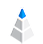# Column Summary - Table of Statistical Information

The column summary or column metadata is a table that contains several statistical and non-statistical information about the variables of the dataset. This summary is about to give a wholesome picture of each column and helps to explore and understand the given dataset including finding outliers, min-max values, dirty data, variation column type, or standard deviation.The following descriptive statistical techniques are automatically calculated by AnswerMiner and represented on the Column Summary table.

## Description

The description of a column can be a piece of detailed information that helps others to understand what is that specific column about. Sometimes the name of the column speaks for itself, but especially for surveys, abbreviations are used in column names.

## Distribution

There is a visualization for each column in the data view and also in the column summary. By that, you can see visually how the values are dispersed.

## Type

AnswerMiner automatically detects the types of columns and based on that suggests visualizations. The following types of columns are used in AnswerMiner: Numeric ID, Unique ID, Logical, Integer, Category, Ordinal, Date, Date-time.

## Unit

As the description gives additional information to a column the measurement unit of the data (currency, amount, weight, etc.) is also useful to give an understanding for others, who are reading the analysis. It is also a standard in statistics that the measurement data is included somehow in the header. The measurement unit of data that you can configure in Column settings at Data view.

## Full range info

The full range info - as the name describes - includes information about all the values included in the given column. In the case of numerical values, the minimum and the maximum values will be displayed. Otherwise, all the categories or date range or unique strings will be shown.

## General range info

The general range info shows the most frequent usual values in the given columns, based on the same logic as the Full-range info does.

## Values with percentage

This column shows each value appearing in the given column and their frequency in percentage.

## Filled Count

This number shows the number of non-empty cells in the given column. This and the filled ratio includes essential information in the exploratory phase.

## Filled Ratio

The filled ratio is the percentage of filled cells compared to all cells inside the column or it is often named as the quality of the column.

## Empty Count

This shows the number of empty cells as reverse information of the Filled Count column.

## Numeric Count

The number of numeric values in a column. This number shows you how many different numeric values are there. If it is less than the number of rows, there are duplicated values or empty cells in your dataset.

## Numeric Ratio

This is the percentage of the numeric values compared to all non-empty cells

## Distinct Count

This is the number of different values in a column. It can quickly help you find out how many elements are in a categorical column.

## Mean

The mean or the average is the sum of numeric values divided by the number of numeric values.

## Median

The median is the middle number of the group when they are ranked in order.

## Mode (most common value)

The mode is the most frequently occurring value.

## Mode count

This number shows the count of the most frequently occurring value - the mode.

## Mode ratio

The mode ratio is the percentage of the most frequently occurring value compared to all non-empty cells.

The table is configurable by sorting the values and add/hide columns and rows. See on the image below.## Min

The min or minimum value is the lowest numeric value in a set of numeric values.

## Max

The max or maximum value is the highest numeric value in a set of numeric values.

## Min to Max

The lowest (min or minimum) and the highest (max or maximum) numeric value in a set of numeric values.

## Standard deviation

The sample standard deviation (SD) is a measure of the amount of variation or dispersion of a set of values (square root of variance).

## Variance

The sample variance is the expectation of the squared deviation of a random variable from its mean (square of standard deviation).

## Population Standard deviation

This is the standard deviation or SD o of the entire population.

## Population Variance

This is the variance or VAR of the entire population.

## Usual Range Low

This is the lower or bottom 10% in a set of numeric values if the numbers are ranked in order.

## Usual Range High

This is the upper or top 10% in a set of numeric values if the numbers are ranked in order.

## Usual Range (middle 80%)

This is the middle 80% of values, which means that the bottom and top 10% are excluded.

The table can be exported as a TSV. It will include all the custom settings like filtering, sorting and included columns and rows set in AnswerMiner.## IQR Low

The IQR Low is the lower value of interquartile range (middle 50% of numbers if ranked in order), lower 25% of numbers.

## IQR High

The IQR High is the upper value of interquartile range (middle 50% of numbers if ranked in order), upper 25% of numbers.

## IQR Range (middle 50%)

The interquartile range or IQR (middle 50% of numbers if ranked in order) is between 25th and 75th percentiles.

## Outlier-free Range Low

The Outlier-free Range Low is the lowerest number that is not an outlier: max(Q1-3*IQR, MaxOfNumbers) where Q1=IQRLow.

## Outlier-free Range High

The Outlier-free Range High is the highest number that is not an outlier: min(Q3+3*IQR, MaxOfNumbers) where Q3=IQRHigh.

## Outlier-free Range

The Outlier-free Range is the full range of numeric values without far outliers (3*IQR rule).

This feature is available in each plan of AnswerMiner. Feel free to try.